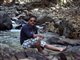# QlikView App Dev

Discussion Board for collaboration related to QlikView App Development.

Announcements
Customer & Partners, DEC. 9, 11 AM ET: Qlik Product & Strategy Roadmap Session: Data Analytics REGISTER NOW
cancel
Showing results for
Did you mean:Not applicable

## Syntax problem i calculation

I´m trying to do this calculation in a list caption:

I have a list that looks like this

2008 1000

2009 1500

the 1000 and 1500 is the frequency

=sum(sales=''2009) - sum (sales='2008')/sum(sales='2009')'100 & '%'

this should give me an increase percentage - but the resultgives me the number - 150268 ???????????

13 RepliesNot applicable
Author

Hi Polschou,

Pls try the below syntax;

Num(((Sum({\$<YEAR={2009}>} Value)-Sum({\$<YEAR={2008}>} Value)) / Sum({\$<YEAR={2009}>} Value)),'#0.00%')

Regards

RajeshNot applicable
Author

Hi Rajesh

sorry it doesnt work - gives no result

I worked a little further with my own and it works now like this

=((sum(sales=''2009) - sum (sales='2008'))/sum(sales='2009'))'100 & '%'

The only problem now is that the result shows the percent like thsi 31,1234567899%

I only want 1 place after the comma - like this 31,1 %Not applicable
Author

Hi,

Eg. Num(<<expression>>, '#0.00%') - take out the 100 & % from the expression.

Regards

RajeshNot applicable
Author

I tried like this

=Num(((sum(sales=''2009) - sum (sales='2008'))/sum(sales='2009'))'100),#0.00% & '%'

but it doesnt workNot applicable
Author

Try this.

=Num(((sum(sales=''2009) - sum (sales='2008'))/sum(sales='2009')),'#0.00%')Not applicable
Author

why don't you use "Number" tab for formatting?.This will solve your problemSpecialist

Try

= sum(If(sales='2009',Value)) - sum (If(sales='2008', Value))/sum(If(sales='2009',Value)) * 100Not applicable
Author

I just get a - so it doesnt work either 🙂Not applicable
Author

ramchander - using number for formatting only changes the formaat in the list not in the captionCommunity Browser